# Wiener-Hopf operator

(diff) ← Older revision | Latest revision (diff) | Newer revision → (diff)

An operator which arises from another operator by compression to a subspace. Given a linear operator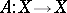and a projection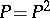onwith range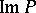, the corresponding Wiener–Hopf operator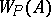is defined as the operator onthat sendsto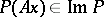. Thus,.

A Wiener–Hopf integral operator formally acts by the rule(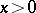) on some space of functions over, say on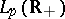(). It may be regarded as the restriction toof a convolution integral operator on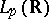. The operatoris bounded if, for example,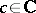and. Many properties ofcan be read off from its symbol. This is the functiongiven byThe operatoris Fredholm (cf. Fredholm operator), i.e. invertible modulo compact operators, if and only if its symbolhas no zeros on the one-point compactification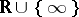(cf. also Aleksandrov compactification) of. In that case the kernel and cokernel dimensions ofare: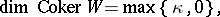where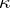is the winding number of the symbolabout the origin. The equation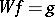can be solved by Wiener–Hopf factorization, which means that one representsin the form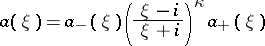such that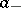and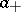extend to analytic functions without zeros in the lower and upper complex half-planes, respectively.

Many interesting operators are Wiener–Hopf integral operators with discontinuous symbols. For example, the Cauchy singular integral operator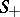(cf. also Singular integral) on(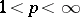),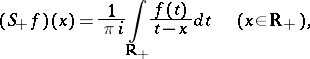can be interpreted as the Wiener–Hopf integral operator with symbol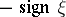. The spectrum ofonis the set of all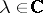at which the line segment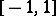is seen at an angle of at least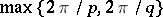, where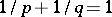.

Wiener–Hopf integral operators with matrix-valued symbols, on finite intervals, or on higher-dimensional domains (including the quarter-plane) have also been extensively studied.

A discrete Wiener–Hopf operator, or a Toeplitz operator (cf. also Toeplitz matrix), is given by a matrix of the formon some sequence space, e.g. on. In this case the symbol is the function on the complex unit circle whose Fourier coefficients constitute the sequence.

There is a rich literature on Wiener–Hopf operators. A good introduction is the classical monograph [a1]; [a2] and [a3] provide an overview of some recent developments.

How to Cite This Entry:
Wiener-Hopf operator. Encyclopedia of Mathematics. URL: http://encyclopediaofmath.org/index.php?title=Wiener-Hopf_operator&oldid=16439
This article was adapted from an original article by A. BÃ¶ttcher (originator), which appeared in Encyclopedia of Mathematics - ISBN 1402006098. See original article